# Block Simulation Network of Elementary Cellular Automata

A network showing how one elementary cellular automaton can emulate another if its states contain only particular blocks

Originator: Stephen Wolfram

All the 88 inequivalent elementary rules are included. This network originally appeared on page 702 of Stephen Wolfram's A New Kind of Science.

## Examples

### Basic Examples

Retrieve the resource:

 In:=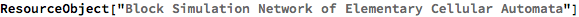Out=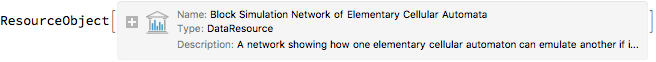Retrieve the default content:

 In:=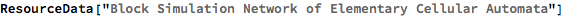Out=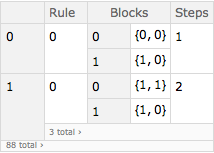Find which rules can be emulated by rule 22:

 In:=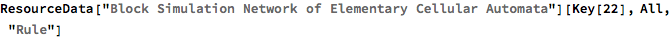Out=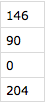Find the details of the emulation of rule 90 by rule 22:

 In:=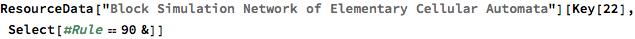Out=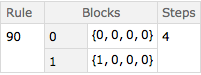Get the blocks:

 In:=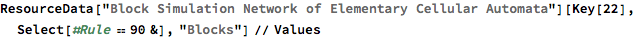Out=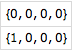Plot them:

 In:=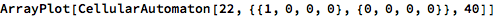Out=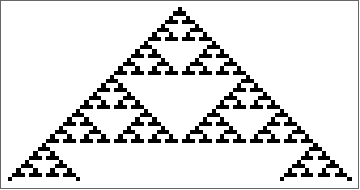Look at every 4th step:

 In:=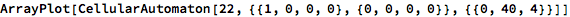Out=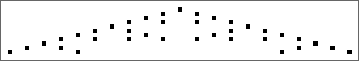Convert the blocks to 0 and 1 respectively:

 In:=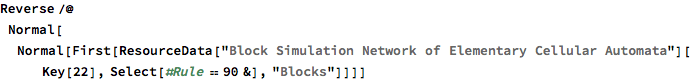Out=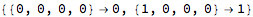In:=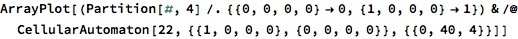Out=Plot the entire simulation network

 In:=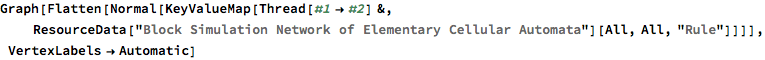Out=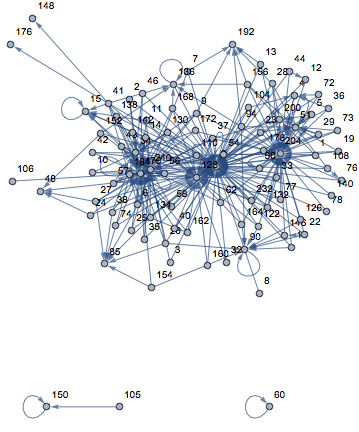Wolfram Research, "Block Simulation Network of Elementary Cellular Automata" from the Wolfram Data Repository (2017) https://doi.org/10.24097/wolfram.05827.data